• +91 9971497814
• info@interviewmaterial.com

# Chapter 7- System of particles and Rotational Motion Interview Questions Answers

### Related Subjects

Question 1 :
.Give the location of the center of mass of a (i) sphere, (ii) cylinder, (iii) ring, and (iv) cube, each of uniform mass density. Does not the center of mass of a body necessarily lie inside the body ?

A sphere. Center of the sphere
A ring. Center of the ring
A cylinder. Middle point on the axis of the cylinder
A cone. At a point distant 1/4th of height of the cone from the base on the axis of the cone.
A cube. Point of intersection of diagonals.
No, center of mass of a ring and bangle lies inside the hollow portions of the ring and bangle.

Question 2 : In the HCl molecule, the separation between the nucleiof the two atoms is about 1.27 A (1 A = 10-10 m).Find the approximate location of the CM of the molecule, given that a chlorineatom is about 35.5 times as massive as a hydrogen atom and nearly all the massof an atom is concentrated in its nucleus.

Answer 2 :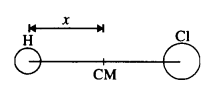Let us choose the nucleus of the hydrogen atom as theorigin for measuring distance.
Mass of hydrogen atom, m1 =1 unit (say)
Mass of chlorine atom, m2 =35.5 units (say)
Now, x1= 0 and x2 = 1.27 A
= 1.27 x 10-10 m
Distance of C.M. of HCl molecule from the origin isgiven by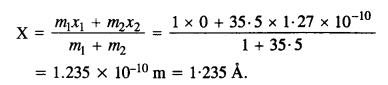Question 3 : A child sits stationary at one end of a long trolleymoving uniformly with a speed V on a smooth horizontal floor. If the child getsup and runs about on the trolley in any manner, what is the speed of C.M. ofthe (trolley + child) system ?

Trolley and the child makes the system. Speed of the C.M. ofthis system remains same irrespective of the movements of the child inside thetrolley in any manner because C.M. is affected by external forces and not bythe internal forces.

Question 4 : Show that the area of the triangle contained betweenthe vectors a and b is one half of the magnitude
of a x b.

Answer 4 :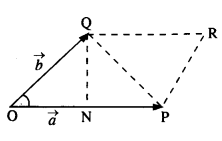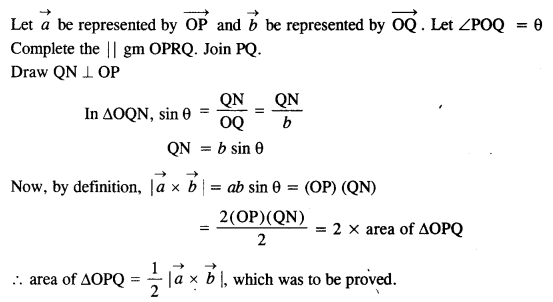Question 5 : Show that a.(b x c) is equal in magnitude to thevolume of the parallelepiped formed on the three vectors a, b and c.

Answer 5 :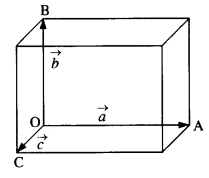Let a parallelepiped be formed on the three vectors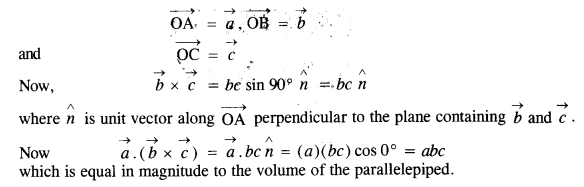Question 6 : Find the components along the x, y, z axes of theangular momentum lofa particle, whose position vector is r withcomponents x, y, z and momentum is with components x, y, z and momentum is p withcomponents px, py and pz. Show that if the particlemoves only in the x-y plane, the angular momentum has only a z-component.

Answer 6 :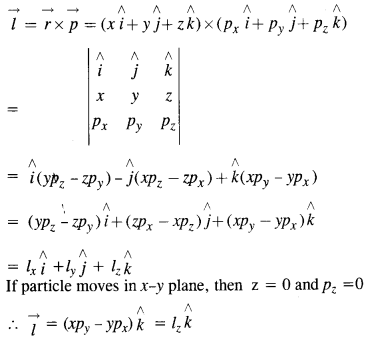Question 7 : Two particles, each of mass m and speed u, travel inopposite directions along parallel lines separated by a distance d. Show thatthe vector angular momentum of the two particle system is the same whatever bethe point about which the angular momentum is taken.

Let at a certain instant twoparticles be at points P andQ, as shown in the following figure.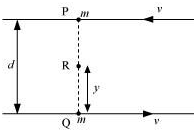Angular momentum of the system about point P: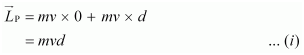Angular momentum of the system about point Q: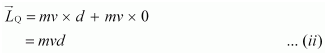Consider a point R, which is at a distance y from point Q, i.e.,

QR = y

PR = d – y

Angular momentum of the system about point R: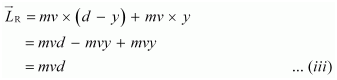Comparing equations (i), (ii),and (iii), we get: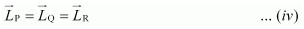We infer from equation (iv) that the angular momentum of a system does notdepend on the point about which it is taken.

Question 8 : A non-uniform bar of weight W is suspended at rest, bytwo strings of negligible  weight as shown in Fig. The angles made by thestrings with the vertical are 36.9° and 53.1° respectively. The bar is 2 mlong. Calculate the distance d of the center of gravity of the bar from itsleft end.

Answer 8 :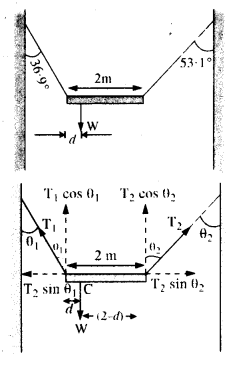As is clear form Fig.,
θ1 = 36.9°,θ2 = 53.1°.
If T1 ,T2 are the tensions inthe two strings, then for equilibrium along the horizontal,
T1  sin θt = T2 sin θ2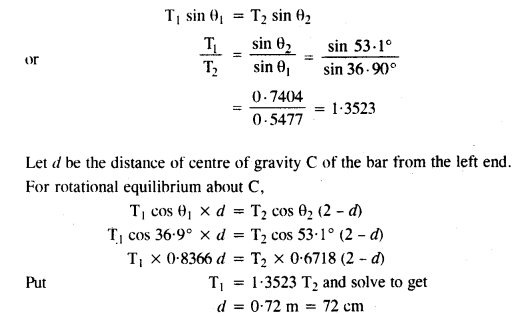Question 9 : A car weighs 1800 kg. The distance between its frontand back axles is 1.8 m. Its center of gravity is 1.05 m behind the front axle.Determine the force exerted by the level ground on each front wheel and eachback wheel.

Answer 9 :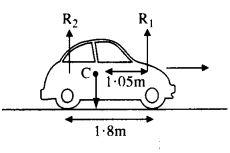Here,     m = 1800 kg
Distance of center of gravity (C) behind the front axle = 1.05 m.
Let R1. R2 be the force exertedby the level ground on front wheels and back wheels. As is clear from fig.,
R1+ R2 = mg = 1800 x 9.8 =17640 N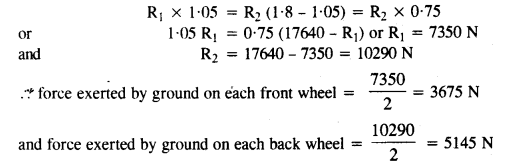Question 10 : (a) Find the moment of inertia of a sphere about atangent to the sphere, given the moment of inertia of the sphere about any ofits diameters to be 2 MR2/5, where M isthe mass of the sphere and R is the radius of the sphere.
(b) What is the moment of inertia of a uniform disc of radius R and mass Mabout an axis
(1) passing through its center and normal to the disc
(2) passing through a point on its edge and normal to the disc ? The moment ofinertia of the disc about any of its diameters is given to be
1 MR2 .

Answer 10 :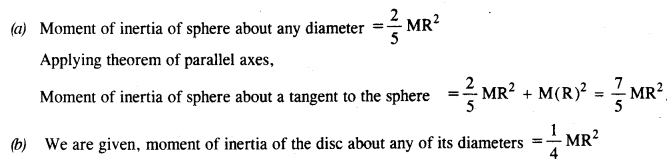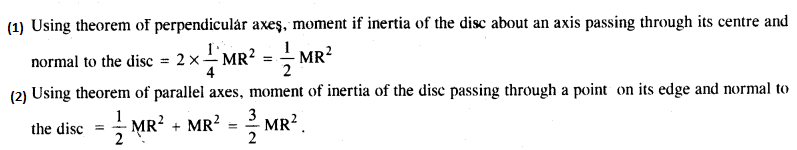Todays Deals### Chapter 7- System of particles and Rotational Motion Contributorskrishan

Name:
Email:

# Latest News# 9000 interview questions in different categories